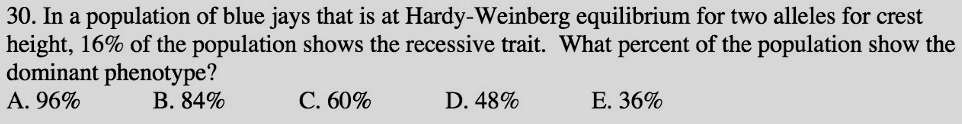# Problem: In a population of blue jays that is at Hardy-Weinberg equilibrium for two alleles forcrest height, 16% of the population shows the recessive trait. What percent of the population show the dominant phenotype?A. 96%B. 84%C. 60%D. 48%E. 36%

###### FREE Expert Solution

If a population is at Hardy-Weinberg equilibrium, it follows the equation for the allele frequency, p + q = 1, where p is the relative frequency of the dominant allele that is present in the population, while q is the relative frequency for the recessive allele. In addition to this, the trait in question would have a constant frequency for the genotypes for the trait.###### Problem Details

In a population of blue jays that is at Hardy-Weinberg equilibrium for two alleles forcrest height, 16% of the population shows the recessive trait. What percent of the population show the dominant phenotype?

A. 96%

B. 84%

C. 60%

D. 48%

E. 36%